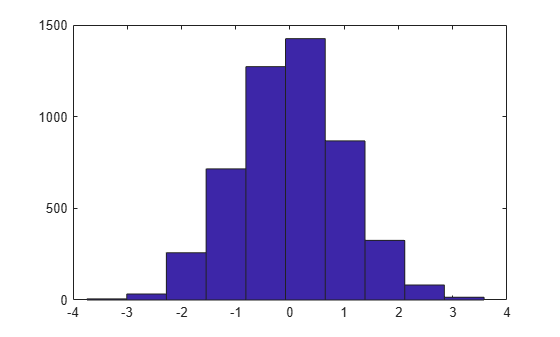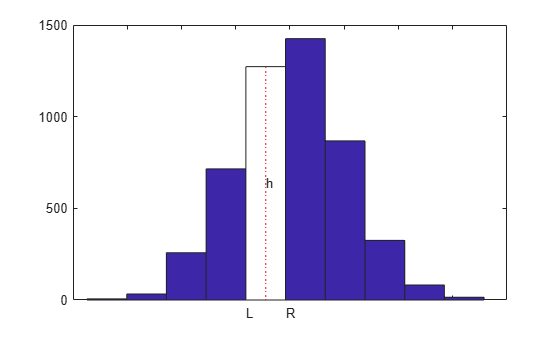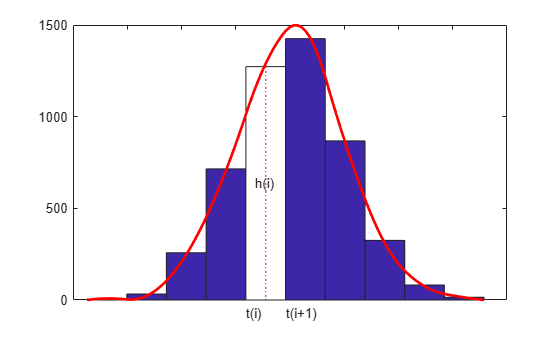Documentation

## Smoothing a Histogram

This example shows how to use spline commands from Curve Fitting Toolbox™ to smooth a histogram.

Here is a histogram of some random values that might represent data that were collected on some measurement.

```y = randn(1,5001); hist(y);```We would like to derive from this histogram a smoother approximation to the underlying distribution. We do this by constructing a spline function `f` whose average value over each bar interval equals the height of that bar.

If `h` is the height of one of these bars, and its left and right edges are at `L` and `R`, then we want the spline `f` to satisfy

` integral {f(x) : L < x < R}/(R - L) = h,`

or, with `F` the indefinite integral of `f`, i.e., `DF = f`,

` F(R) - F(L) = h*(R - L).`

```[heights,centers] = hist(y); hold on ax = gca; ax.XTickLabel = []; n = length(centers); w = centers(2)-centers(1); t = linspace(centers(1)-w/2,centers(end)+w/2,n+1); p = fix(n/2); fill(t([p p p+1 p+1]),[0 heights([p p]),0],'w') plot(centers([p p]),[0 heights(p)],'r:') h = text(centers(p)-.2,heights(p)/2,' h'); dep = -70; tL = text(t(p),dep,'L'); tR = text(t(p+1),dep,'R'); hold off```So, with `n` the number of bars, `t(i)` the left edge of the `i`-th bar, `dt(i)` its width, and `h(i)` its height, we want

` F(t(i+1)) - F(t(i)) = h(i) * dt(i), for i = 1:n,`

or, setting arbitrarily `F(t(1))` = 0,

` F(t(i)) = sum {h(j)*dt(j) : j=1:i-1}, for i = 1:n+1.`

```dt = diff(t); Fvals = cumsum([0,heights.*dt]);```

Add to this the two end conditions `DF(t(1)) = 0 = DF(t(n+1))`, and we have all the data we need to get `F` as a complete cubic spline interpolant.

`F = spline(t, [0, Fvals, 0]);`

The two extra zero values in the second argument indicate the zero endslope conditions.

Finally, the derivative, `f = DF`, of the spline `F` is the smoothed version of the histogram.

```DF = fnder(F); % computes its first derivative h.String = 'h(i)'; tL.String = 't(i)'; tR.String = 't(i+1)'; hold on fnplt(DF, 'r', 2) hold off ylims = ylim; ylim([0,ylims(2)]);```